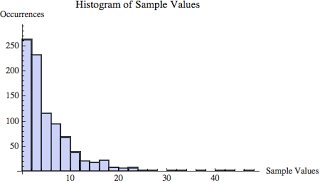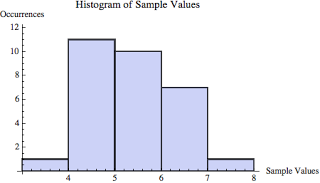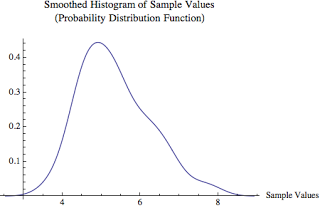## Wanna Bet? Cool Facts About The Central Limit Theorem.

Posted on 28-Feb-2012 by Craig Shallahamer, craig@orapub.com

### Wanna Bet?Suppose there is a room of 75 fanatical Oracle Database Administrators. (They may even look like the guys in this picture!) I randomly pick 25 of them and ask them to randomly divide up into 5 groups of 5 each.

Then I ask each group to compute the average age for their group and give me the results. The averages are 38.2, 34.7, 41.2, 43.9, and 42.1. I do a little math but don't say anything.

I then look to the remaining 50 DBAs, ask them to break up into 10 groups of 5 each, compute their group's average age, write their result on a piece of paper, and without showing me, place the paper face down on the table before me.

I take a deep breath, reach into my pocket, take out a crisp new \$100 bill, and place it on the table next to the ten pieces of paper. I then proclaim that if anyone is willing to match my \$100 bet, I will turn over the ten pieces of paper in front of me and bet them that written on nine out of the ten are values between 32.8 and 47.2. The range doesn't spook the group, but the 9 out of 10 visibly does.

No one says a word... I start breathing again.

But just for fun, I slowly peal back each piece of paper one by one exposing their values: 42.4, 35.9, 49.7 (oh oh...), 46.1, 45.3, 40.0, 43.0, 39.3, 35.5, and 42.1. So 9 out of the 10 were between 32.8 and 47.2. Was I lucky? No. Could I have lost my \$100? Yes, but it was unlikely and why the saying, "Two out of three?" came from I suppose.

So how did I know the odds were in my favor even with less than a 14 year spread? Believe it or not, the principle demonstrated is this: If you divide up a population into sample sets and calculate the mean of each sample set, the distribution of the means will be normal. This normality characteristic allows us to make bold "plus or minus" statements... perhaps with a \$100 bill involved. Can't wait until Collaborate/IOUG 2012 in Las Vegas!

While this trick is pretty cool, in my view, the really cool part is it will work using years of Oracle experience, number of kids the DBA has, and size of the DBA's IT department. Or more boring figures like Oracle Database wait event times, SQL elapsed times, and SQL arrival rates.

### The fine print.

The distribution being normal tells us that 90% of the samples are between plus or minus 1.645 times the standard deviation from the average. In my example, the average of the initial five sample sets is 40.0, the standard deviation is 3.6, so the "minus" 90% bound is 34.1, and the "plus" 90% bound is 46.0. But those aren't the bounds I used in the challenge!

You may have noticed my "wanna bet" range was not 34.1 and 46.0 (90% range) but rather between 32.8 and 47.2. I needed some insurance because I'm using less than 30 sample values so the math to determine the upper and lower bounds is slightly different. So to hedge my bet, I used the 95% level instead of 90%, which is 2 times the standard deviation, not 1.645. This is why and how I used the bounds of 43.8 and 47.2 (95% range).

If you really dig into this, at its core this is about the central limit theorem. This also indirectly relates to what is known as the standard error, which I'll save for another posting.

What does this have to do with our performance analysis work? As I explain in more detail at the end of the Demonstration On A Larger Scale section, this posting touches on the very core of making "plus and minus" statements. As I have blogged previously, using only the average leaves a lot to the imagination and can cause miscommunication. The "plus and minus" presented in this posting is central to understanding and making "plus and minus" predictive analysis statements, but with an interesting twist.

If you want to follow exactly how I did this, you'll want to download the Mathematica notepad in either PDF or native format. It's pretty much top down and you'll see all statistics including the histograms.

### Demonstration On A Larger Scale

I was never one to grasp mathematical proofs or believe all that I read. And especially with a flimsy "test" using 15 sample sets of 5 values each. For me, I need to visually see repeated examples before I began to trust claims like I've stated above. I could have selected one very convincing example above to demonstrate my points... and I did! Why? Because I wanted to keep the number of values very small to reduce confusion. But to see if the above claims are really true, we need a much more robust test. And that's what I've done and will describe below.

LOTS of notes and all the information (data, visuals, statistics) used in this example is available for download in the Mathematica notepad in PDF or the native format.

Step 1. Create a sample set.

I created a 900 value sample set based on the exponential distribution with an average of 5.0. The actual mean of the 900 values is 5.343. (The means don't match exactly because I'm randomlly pulling my samples from a mathematical formula or distribution.) I choose the exponential distribution because it is clearly (both visually and numerically) not normally distributed and will confirm some of the wild claims I'll make.The above histogram is composed of the raw 900 values. Clearly the values are not normally distributed but look very exponentially distributed (because they are). In fact, the normality test p-value is 0.00000 and we need a 0.05 for the data to likely be normal.

Step 2. From the parent sample set, create a number of child sample sets.

I wrote a program in Mathematica to sequentially pull from the parent sample set, 30 sample sets each containing 30 sample values. So every one of the 900 population values reside in one of the 30 child sample sets. I'm not going to post all the samples here, but they are included in the Mathematica file (both pdf and native).

Detail: To ensure I didn't use a sample value twice, I started pulling from the population set sequentially. A variable sets the starting sample place, which in this example is the sixth. To ensure I didn't try to pull beyond the end of the population sample set, I simply joined the initial 900 sample set to itself.

Step 3. Calculate the mean of each child sample set.

Here are means for the 30 child sample sets that were created in Step 2 above. There is one mean for each of the 30 child sample sets.

``{6.35511, 4.91329, 3.7737, 5.27418, 6.52658, 7.68304, 4.4319,6.09553, 4.64664, 6.10894, 4.422, 5.21073, 4.33155, 6.78821, 5.38437, 5.38456, 5.80179, 4.60562, 4.87516, 6.77315, 5.38634, 5.55096, 5.43312, 4.48588, 4.75642, 5.06397, 4.43674, 4.72971, 5.00164, 6.06055} ``

The mean of the parent sample set and the mean of the sub sample sets are the same!

According to the central limit theorem, the mean of the parent sample set and the mean of the child sample sets are the same. The parent sample set mean is 5.343 (see Step 1) and mean of the 30 child sample sets is 5.343. Not bad! Actually, if you try this yourself once the number of sample sets is greater than 20 and all the population values are included in the child sample sets, their means will be pretty darn close.

Is the distribution of child sample set means normal?

According to the central limit theorem, a distribution of means (e.g., the means from our 30 child sample sets) is normal. Below is a histogram based on our 30 means.Based on the same 30 means, below is a smoothed histogram. Sometimes it is much easier to see the normality when using a smoothed histogram.These histograms are called the sampling distribution of the mean. The standard histogram doesn't look perfectly normal, but statistically it is! I performed a normality check and the p-value is 0.185 and we only needed a value greater than 0.05 for the values to likely be normally distributed. This is awesome that a distribution of sample means is normal... because now we can make some very bold statements about these sample values (i.e., the means from our 30 child sample sets).

Using the normal distribution claim.

Because the distribution of the child sample set means is normal, 95% of the child sample set means will be within two standard deviations of the mean (the mean of our 30 child sample sets). The mean of the child sample sets (of mean values) is 5.343 and the standard deviation is 0.892. Therefore, 95% of the 30 child sample sets means will be between 5.343 +/- 2*0.892 which is also 5.34 +/-1.78 which is from 3.56 to 7.12, 95% of the time. Statistically this is true, but is it really true?

If you look back to Step 3, where the 30 means are listed, you will notice that 29 of the means are between 3.56 and 7.12, which is 97%. How cool is that!

Now let's scale it up!

Just for fun I increased the sample set size from 900 to 8100, which resulted in 90 child sample sets, each with 90 sample values. I then reran the numbers. The population mean and the mean of the 90 child sample set means is also 5.028.(!!) Not only does the histogram look normal, but the normality test resulted in a p-value of 0.62, which is above our 0.050 threshold indicating the sample set is likely to be normal. How many samples where within two times the standard deviation? Hopefully at least 95% and in this situation, 98% or 88 out of 90 where within the boundaries!

### Making This Practical

All this math is actually very practical when you want to describe a set of numbers with more than simply the average. As I've written about previously, the word average invokes the possibility of an unlimited number of possible perceptions of the situation. Simply providing the "plus and minus" and perhaps a histogram can significantly improve communication.

But the challenge is, many times our data is not normal. And as I've demonstrated, when the data is normal we can make some very bold (well... not that bold actually) claims.

However, demonstrating the data is not normal is valuable because it breaks the typical picture people have in the minds when we talk about, for example, the average SQL statement run time or the average wait event time. Knowing a set of data is not normal is valuable because it improves our understanding of the data.

But what I really like about this is regarding making predictive analysis statements. When making a prediction, it's not enough to only supply the average. Part of developing a robust predictive model is understanding its precision and usefulness. One of the ways to do this is understanding its "plus and minus." But if the underlying data is not normal, the "plus and minus" doesn't really help much. However, when undemanding a predictive model's precision, we are describing the average error (and the key word is average). If we have a nice set of data (there is sooo much I can say here, but won't) then the distribution of the error will be normal allowing us to make bold "plus and minus" statements about the precision of our forecasting model. Really powerful stuff.

Sales pitch: If you're interested forecasting, you'll be into my Forecasting Oracle Performance book and my Oracle Forecasting and Predictive Analysis class.

### Summary

This posting took me many, many hours to create. I started with the central limit theorem and then tried to make it work using real numbers. That was the Demonstrating On A Larger Scale part of this posting. But to make this a little more fun and interesting, I wanted to turn it into a story. The "wanna bet" introduction was the result. I'm hoping you learned something of value and if you're a statistical master you have seen the theory play out using real numbers, perhaps, for the first time.

Summarizing the key take-aways, I'd distill them into these five points:

1. There is value in knowing if data is or is not normally distributed. If we understand the distribution, we can make make bold statements about the data. Plus it helps everyone better understand what the "average" value implies.

2. If a data set is normally distributed we can make bold "plus and minus" statements. For example, 95% of the samples are within plus or minus two standard deviations of the mean.

3. The mean of the parent sample set and the mean of the child sample set means are the same. (And I thought saying, "This is the LRU end of the LRU." confused people!)

4. A distribution of means is normal REGARDLESS of the parent distribution. I hope this is crystal clear in your mind. This enables point 5.

5. We can use bold normality statements when describing forecasting model precision (i.e., error).